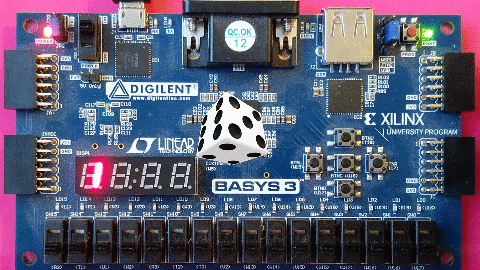Thu. Sep 28th, 2023

# Digital Dice Roller in HLSAbstract: Designing digital systems with HLS is fun and easy. In this project, I am going to implement a digital dice roller on Basys3 board using HLS. If you are interested in HLS and want to know more about HLS and its techniques, please refer here or here.

In order to implement a dice roller on an FPGA using HLS, we should describe three tasks:
1- get the player command,
2- run a random number generator to generate a number between 1 and 6,
3- display the generated number.
For the first task, I am using two push-buttons available on the Basys 3 evaluation board. An LFSR can generate a pseudo-random number. Finally, two 7-segments are used to display the generated numbers for two players.

The following figure shows the push-buttons and 7-segment displays.

The following code represents an LFSR with the equation of$x^{32}+x^{22}+x^2+x+1$

```ap_uint<32> pseudo_random(ap_uint<32> seed, bool load) {
#pragma HLS INLINE off
static ap_uint<32> lfsr;

lfsr = seed;
bool b_32 = lfsr.get_bit(32-32);
bool b_22 = lfsr.get_bit(32-22);
bool b_2 = lfsr.get_bit(32-2);
bool b_1 = lfsr.get_bit(32-1);
bool new_bit = b_32 ^ b_22 ^ b_2 ^ b_1;
lfsr = lfsr >> 1;
lfsr.set_bit(31, new_bit);

return lfsr;

}```

The HLS top-function that receivesthe players’ commands and displays the random numbers are as follows.

```void dice_roller(
ap_uint<8>  &seven_segment_data,
ap_uint<4>  &seven_segment_enable,
bool         first_player,
bool         second_player

) {
#pragma HLS INTERFACE ap_ctrl_hs port=return
#pragma HLS INTERFACE ap_none port=second_player
#pragma HLS INTERFACE ap_none port=first_player
#pragma HLS INTERFACE ap_none port=seven_segment_enable
#pragma HLS INTERFACE ap_none port=seven_segment_data

static int state   = 0;
static ap_uint<8>  segment_data = svn_sg_code;
static ap_uint<4>  segment_enable = 0b1111;

if (state == 0) {
pseudo_random(11, 1);
state = 1;
} else {
pseudo_random(0, 0);
}

if (first_player == 1) {
ap_uint<32> r = pseudo_random(0, 0);
ap_uint<4> dice      = r%6 + 1;
segment_data   = svn_sg_code[dice];
segment_enable = 0b1110;

} else if (second_player == 1) {
ap_uint<32> r = pseudo_random(0, 0);
ap_uint<4> dice      = r%6 + 1;
segment_data   = svn_sg_code[dice];
segment_enable = 0b0111;
} else {
ap_uint<32> r = pseudo_random(0, 0);
}

seven_segment_data   = segment_data;
seven_segment_enable = segment_enable;
}```

After synthesising the code and generating the corresponding RTL-IP, we should perform the logic synthesis in Vivado.